# Maths - Symmetric Groups

### Symmetric group

The group of all permutations is the symmetric group. The term permutation group is usually used for a subgroup of the symmetric group.

The number of possible ways to order the set is n factorial as we can see from this table:

number of elements of a set possible ways to order set
1 1
2 2
3 6
4 24
n !n

There is a 1:1 correspondence between the ordering of the set and mappings between the set and itself. For instance, in the table below, under reordering we show the elements of the set being rearranged and under mapping we show each element being mapped to another element:

Reordering Mapping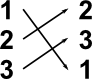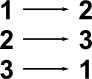But these are both ways of denoting the same set, i.e. each element of the set maps to another (or possibly same) element of the set.

We can assign a letter to each of these mappings:

 permutation cycle notation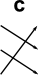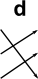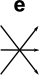(1)(2)(3) (1)(2 3) (1 2)(3) (1 2 3) (3 2 1) (1 3)(2)

These letters then become the elements of a set of permutations. We can then work out the result of the composition of these permutations:#### o#### =To be consistent with other literature on this, we need to reverse the order of the functions, that is the right hand function is applied first then the left hand function.

We can draw up a complete table of the composition operation which defines this example completely:

row o col i a b c d e
iabcdeWe can also work out the inverse of each permutation:

 x x-1The number of possible ways to order the set is n factorial as we can see from this table:

number of elements of a set possible ways to order set
1 1
2 2
3 6
4 24
n !n

## Generating a Alternating Group using a Program

We can use a computer program to generate these groups, here I have used Axiom/FriCAS which is described here.

```s1 := symmetricGroup(1)

```
<1>
```

Type: PermutationGroup(Integer)
toTable()\$toFiniteGroup(s1,1)

```
 a a a a
```

Type: Table(2)
permutationRepresentation(s1,1)

```
[
 1
]
```

Type: List(Matrix(Integer))
s2 := symmetricGroup(2)

```
<(1 2)>
```

Type: PermutationGroup(Integer)
toTable()\$toFiniteGroup(s2,1)

```
 i a a i
```

Type: Table(2)
permutationRepresentation(s2,2)

```
[
 0 1 1 0
]
```

Type: List(Matrix(Integer))
s3 := symmetricGroup(3)

```
<(1 2 3),(1 2)>
```

Type: PermutationGroup(Integer)
toTable()\$toFiniteGroup(s3,1)

```
 i a b aa ab ba a aa ab i ba b b ba i ab aa a aa i ba a b ab ab b a ba i aa ba ab aa b a i
```

Type: Table(6)
permutationRepresentation(s3,3)

```
[
 0 0 1 1 0 0 0 1 0
,
 0 1 0 1 0 0 0 0 1
]
```

Type: List(Matrix(Integer))
s4 := symmetricGroup(4)

```
<(1 2 3 4),(1 2)>
```

Type: PermutationGroup(Integer)
order(s4)

```
24
```

Type: PositiveInteger
permutationRepresentation(s4,4)

```
[
 0 0 0 1 1 0 0 0 0 1 0 0 0 0 1 0
,
 0 1 0 0 1 0 0 0 0 0 1 0 0 0 0 1
]
```

Type: List(Matrix(Integer))
s5 := symmetricGroup(5)

```
<(1 2 3 4 5),(1 2)>
```

Type: PermutationGroup(Integer)
order(s5)

```
120
```

Type: PositiveInteger
permutationRepresentation(s5,5)

```
[
 0 0 0 0 1 1 0 0 0 0 0 1 0 0 0 0 0 1 0 0 0 0 0 1 0
,
 0 1 0 0 0 1 0 0 0 0 0 0 1 0 0 0 0 0 1 0 0 0 0 0 1
]
```

Type: List(Matrix(Integer))
s6 := symmetricGroup(6)

```
<(1 2 3 4 5 6),(1 2)>
```

Type: PermutationGroup(Integer)
order(s6)

```
720
```

Type: PositiveInteger
permutationRepresentation(s6,6)

```
[
 0 0 0 0 0 1 1 0 0 0 0 0 0 1 0 0 0 0 0 0 1 0 0 0 0 0 0 1 0 0 0 0 0 0 1 0
,
 0 1 0 0 0 0 1 0 0 0 0 0 0 0 1 0 0 0 0 0 0 1 0 0 0 0 0 0 1 0 0 0 0 0 0 1
]
```
Type: List(Matrix(Integer))
(19) ->
```

where:

• The points of the permutation are numbered 1..n
• The elements of the group are named: "i" for the identity, single letters "a","b"... for the generators, and products of these.
• numbers in brackets are points of permutations represented in cyclic notation.
• The permutation is represented by a set of comma seperated permutations in angle brackets like this: <(1 2)(3 4),(1 2 3)>
• non-changing elements of the permutation are ommited so the above case is equivalent to: <(1 2)(3 4),(1 2 3)(4)>
• attempting to produce a symmetric group with less than 3 elements does not produce a valid result.
• The Axiom/FriCAS program can't work in terms of the Cayley table, so I have added my own code to do this.

## Young Group

The direct product of the symmetric groups given by the parts of the partition λSymmetry and the Monster - This is a popular science type book which traces the history leading up to the discovery of the largest symmetry groups.